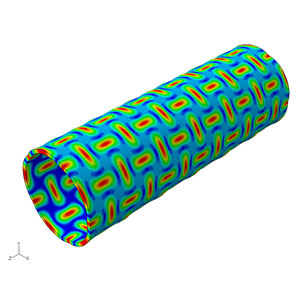Subscribe to our newsletter to receive the latest news and events from TWI:

# What is Finite Element Analysis (FEA)?

Finite element analysis (FEA) is the process of simulating the behaviour of a part or assembly under given conditions so that it can be assessed using the finite element method (FEM). FEA is used by engineers to help simulate physical phenomena and thereby reduce the need for physical prototypes, while allowing for the optimisation of components as part of the design process of a project.

##FEA uses mathematical models to understand and quantify the effects of real-world conditions on a part or assembly. These simulations, which are conducted via specialised software, allow engineers to locate potential problems in a design, including areas of tension and weak spots.

With the use of mathematics it is possible to understand and quantify structural or fluid behaviour, wave propagation, thermal transport and other phenomena.

Most of the processes can be described using partial differential equations (PDEs), but these complex equations need to be solved in order for parameters such as stress and strain rates to be estimated. FEA allows for an approximate solution to these problems.FEA is the basis of modern software simulation software, with the results usually shown on a computer-generated colour scale.

While some theories state that FEA has its roots in the 16th century work of Euler, the earliest mathematical papers directly detailing the technique date back to Schellbach’s work of 1851. FEA was developed further by engineers from different industries around the world in order to solve a large number of structural mechanics problems, primarily in civil engineering and aerospace. The first development of FEA for real world applications began in the mid-1950s and was further developed over the next few decades.

## How Does Finite Element Analysis Work?

The simulations used in FEA are created using a mesh of millions of smaller elements that combine to create the shape of the structure that is being assessed. Each of these small elements is subjected to calculations, with these mesh refinements combining to produce the final result of the whole structure.

These approximate calculations are usually polynomial, with interpolations occurring across the small elements, meaning that values can be determined at some but not all points. The points where the values can be determined are called nodal points and can usually be found at the boundary of the element.

### Partial Differential Equations

As mentioned above, finite element analysis is used to solve partial differential equations, but some PDEs are more suitable.